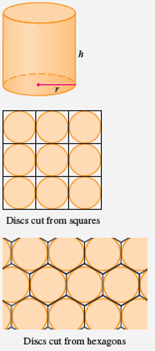# Sec.4.7 - The shape of a can

Applied Project 1 in Sec.4.7, Calculus by Stewart
Chinese version: 罐頭的形狀

Given a cylindrical can with fixed volume $v$, we want to find the height $h$ and radius $r$ that minimize the cost of metal to make the can.

If we disregard any waste metal in the manufacturing process, then the problem is to minimize the surface area of the cylinder. It can be found that the solution of this problem is $h=2r$; this is, the height should be the same as the diameter.

But, for most of the cans in the supermarket, the height is usually greater than the diameter and the ratio $h/r$ varies from $2$ up to about $3.8$. Let’s see if we can explain this phenomenon.Question 1: The material for the cans is cut from sheets of metal. The cylindrical sides are formed by bending rectangles; these rectangles are cut from the sheet with little or no waste.

But if the top and bottom discs are cut from squares of side $2r$, this leaves considerable waste metal, which may be recycled but has little or no value to the can makers. If this is the case, find the ratio $h/r$ for which the amount of metal used is minimized.

$$\frac{h}{r} = \frac{8}{\pi}\approx 2.55$$

Question 2: A more efficient packing of the discs is obtained by dividing the metal sheet into hexagons and cutting the circular lids and bases from the hexagons. If this strategy is adopted, find the ratio $h/r$ for which the amount of metal used is minimized.

$$\frac{h}{r} = \frac{4\sqrt{3}}{\pi}\approx 2.21$$

Question 3: In addition to the cost of the metal, we need to incorporate the manufacturing of the can into the cost.

• Assuming that most of the expense is incurred in joining the sides.

For a cylindrical can, we need to bend a rectangle and joinging the sides together (with length $h$) to make the cylindrical shell. Also we need to join the two discs to the shell (each disc with length $2\pi r$). So the cost in joining is $k(h + 4\pi r)$ where $k$ is the reciprocal of the length that can be joined for the cost of one unit area of metal.

If we cut the discs from hexagons as in Question 2, then the total cost consists of the cost of the metal and the cost in joinging the sides, that is proportional to $4\sqrt{3}r^2 + 2\pi r h + k(4\pi r + h)$.

with the minimum metal used,

$$\frac{h}{r} = \frac{4\sqrt{3}r + 2k\pi}{\pi r + k}.$$

Question 4: What happened when a can is large or joining is cheap, and what happened when the can is small or joining is costly?

For a cylinder with fixed volume $v$:

• When joining is cheap, $0<k\ll 1$, we have $$\frac{h}{r} = \frac{4\sqrt{3}r + 2k\pi}{\pi r + k} \to \frac{4\sqrt{3}}{\pi}\approx 2.21.$$
• When joining is costly, $k\gg 1$, we have $$\frac{h}{r} = \frac{4\sqrt{3}r + 2k\pi}{\pi r + k} \to 2\pi\approx 6.28.$$

If $k$ is fixed, we need to firstly find the relation between the volume $v$ and the ratio $h/r$.

For convenience of notation we define $R=h/r$. Then we rewrite $r$ as a function of $v$ and $R$ as $$r = \sqrt{\frac{v}{\pi R}}.$$ With careful calculation we obtain $$\frac{\sqrt{v}}{k} = \frac{\sqrt{\pi R}(2\pi - R)}{\pi R - 4\sqrt{3}}.$$

• When a can is large, $v\gg 1$, we have that the denominator of the term of the right hand side should approaches $0$, that is, $$\pi R - 4\sqrt{3} \to 0 \Longrightarrow \frac{h}{r} = R \to \frac{4\sqrt{3}}{\pi}\approx 2.21.$$
• When a can is small, $0<v\ll 1$, we have that the numerator of the term of the right hand side should approaches $0$, that is, $$2\pi - R \to 0 \Longrightarrow \frac{h}{r} = R \to 2\pi\approx 6.28.$$